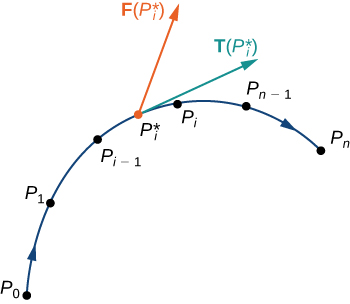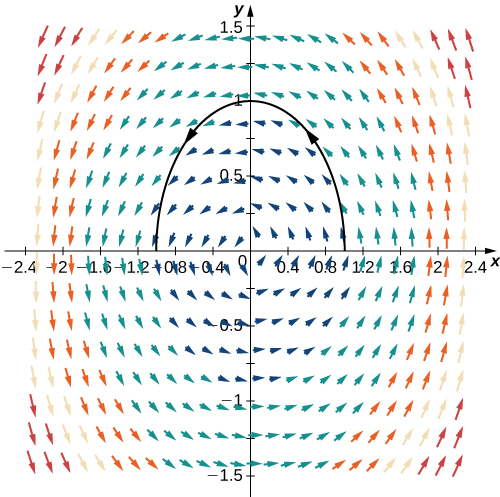# 6.2 Line integrals  (Page 5/20)

 Page 5 / 20

Let $\text{r}\left(t\right)$ be a parameterization of C for $a\le t\le b$ such that the curve is traversed exactly once by the particle and the particle moves in the positive direction along C . Divide the parameter interval $\left[a,b\right]$ into n subintervals $\left[{t}_{i-1},{t}_{i}\right],0\le i\le n,$ of equal width. Denote the endpoints of $\text{r}\left({t}_{0}\right),\text{r}\left({t}_{1}\right)\text{,…},\text{r}\left({t}_{n}\right)$ by ${P}_{0}\text{,…},{P}_{n}.$ Points P i divide C into n pieces. Denote the length of the piece from P i−1 to P i by $\text{Δ}{s}_{i}.$ For each i , choose a value ${t}_{i}^{*}$ in the subinterval $\left[{t}_{i-1},{t}_{i}\right].$ Then, the endpoint of $\text{r}\left({t}_{i}^{*}\right)$ is a point in the piece of C between ${P}_{i-1}$ and P i ( [link] ). If $\text{Δ}{s}_{i}$ is small, then as the particle moves from ${P}_{i-1}$ to ${P}_{i}$ along C , it moves approximately in the direction of $\text{T}\left({P}_{i}\right),$ the unit tangent vector at the endpoint of $\text{r}\left({t}_{i}^{*}\right).$ Let ${P}_{i}^{*}$ denote the endpoint of $\text{r}\left({t}_{i}^{*}\right).$ Then, the work done by the force vector field in moving the particle from ${P}_{i-1}$ to P i is $\text{F}\left({P}_{i}^{*}\right)·\left(\text{Δ}{s}_{i}\text{T}\left({P}_{i}^{*}\right)\right),$ so the total work done along C is

$\sum _{i=1}^{n}\text{F}\left({P}_{i}^{*}\right)·\left(\text{Δ}{s}_{i}\text{T}\left({P}_{i}^{*}\right)\right)=\sum _{i=1}^{n}\text{F}\left({P}_{i}^{*}\right)·\text{T}\left({P}_{i}^{*}\right)\text{Δ}{s}_{i}.$Curve C is divided into n pieces, and a point inside each piece is chosen. The dot product of any tangent vector in the i th piece with the corresponding vector F is approximated by F ( P i * ) · T ( P i * ) .

Letting the arc length of the pieces of C get arbitrarily small by taking a limit as $n\to \infty$ gives us the work done by the field in moving the particle along C . Therefore, the work done by F in moving the particle in the positive direction along C is defined as

$W={\int }_{C}\text{f}·\le \text{T}ds,$

which gives us the concept of a vector line integral.

## Definition

The vector line integral    of vector field F along oriented smooth curve C is

${\int }_{C}\text{F}·\text{T}ds=\underset{n\to \infty }{\text{lim}}\sum _{i=1}^{n}\text{F}\left({P}_{i}^{*}\right)·\text{T}\left({P}_{i}^{*}\right)\text{Δ}{s}_{i}$

if that limit exists.

With scalar line integrals, neither the orientation nor the parameterization of the curve matters. As long as the curve is traversed exactly once by the parameterization, the value of the line integral is unchanged. With vector line integrals, the orientation of the curve does matter. If we think of the line integral as computing work, then this makes sense: if you hike up a mountain, then the gravitational force of Earth does negative work on you. If you walk down the mountain by the exact same path, then Earth’s gravitational force does positive work on you. In other words, reversing the path changes the work value from negative to positive in this case. Note that if C is an oriented curve, then we let − C represent the same curve but with opposite orientation.

As with scalar line integrals, it is easier to compute a vector line integral if we express it in terms of the parameterization function r and the variable t . To translate the integral ${\int }_{C}\text{F}·\text{T}ds$ in terms of t , note that unit tangent vector T along C is given by $\text{T}=\frac{{r}^{\prime }\left(t\right)}{‖{r}^{\prime }\left(t\right)‖}$ (assuming $‖{r}^{\prime }\left(t\right)‖\ne 0\right).$ Since $ds=‖{r}^{\prime }\left(t\right)‖dt,$ as we saw when discussing scalar line integrals, we have

$\text{F}·\text{T}ds=\text{F}\left(\text{r}\left(t\right)\right)·\frac{{r}^{\prime }\left(t\right)}{‖{r}^{\prime }\left(t\right)‖}‖{r}^{\prime }\left(t\right)‖dt=\text{F}\left(\text{r}\left(t\right)\right)·{r}^{\prime }\left(t\right)dt.$

Thus, we have the following formula for computing vector line integrals:

${\int }_{C}\text{F}·\text{T}ds={\int }_{a}^{b}\text{F}\left(\text{r}\left(t\right)\right)·{r}^{\prime }\left(t\right)dt.$

Because of [link] , we often use the notation ${\int }_{C}\text{F}·d\text{r}$ for the line integral ${\int }_{C}\text{F}·\text{T}ds.$

If $\text{r}\left(t\right)=⟨x\left(t\right),y\left(t\right),z\left(t\right)⟩,$ then d r denotes vector $⟨{x}^{\prime }\left(t\right),{y}^{\prime }\left(t\right),{z}^{\prime }\left(t\right)⟩.$

## Evaluating a vector line integral

Find the value of integral ${\int }_{C}\text{F}·d\text{r},$ where $C$ is the semicircle parameterized by $\text{r}\left(t\right)=⟨\text{cos}\phantom{\rule{0.2em}{0ex}}t,\text{sin}\phantom{\rule{0.2em}{0ex}}t⟩,$ $0\le t\le \pi$ and $\text{F}=⟨\text{−}y,x⟩.$

We can use [link] to convert the variable of integration from s to t . We then have

$\text{F}\left(\text{r}\left(t\right)\right)=⟨\text{−}\text{sin}\phantom{\rule{0.2em}{0ex}}t,\text{cos}\phantom{\rule{0.2em}{0ex}}t⟩\phantom{\rule{0.2em}{0ex}}\text{and}\phantom{\rule{0.2em}{0ex}}{r}^{\prime }\left(t\right)=⟨\text{−}\text{sin}\phantom{\rule{0.2em}{0ex}}t,\text{cos}\phantom{\rule{0.2em}{0ex}}t⟩.$

Therefore,

$\begin{array}{cc}\hfill {\int }_{C}\text{F}·d\text{r}& ={\int }_{0}^{\pi }⟨\text{−}\text{sin}\phantom{\rule{0.2em}{0ex}}t,\text{cos}\phantom{\rule{0.2em}{0ex}}t⟩·⟨\text{−}\text{sin}\phantom{\rule{0.2em}{0ex}}t,\text{cos}\phantom{\rule{0.2em}{0ex}}t⟩dt\hfill \\ & ={\int }_{0}^{\pi }{\text{sin}}^{2}t+{\text{cos}}^{2}tdt\hfill \\ & ={\int }_{0}^{\pi }1dt=\pi .\hfill \end{array}$This figure shows curve r ( t ) = ⟨ cos t , sin t ⟩ , 0 ≤ t ≤ π in vector field F = ⟨ − y , x ⟩ .

explain and give four Example hyperbolic function
The denominator of a certain fraction is 9 more than the numerator. If 6 is added to both terms of the fraction, the value of the fraction becomes 2/3. Find the original fraction. 2. The sum of the least and greatest of 3 consecutive integers is 60. What are the valu
1. x + 6 2 -------------- = _ x + 9 + 6 3 x + 6 3 ----------- x -- (cross multiply) x + 15 2 3(x + 6) = 2(x + 15) 3x + 18 = 2x + 30 (-2x from both) x + 18 = 30 (-18 from both) x = 12 Test: 12 + 6 18 2 -------------- = --- = --- 12 + 9 + 6 27 3
Pawel
2. (x) + (x + 2) = 60 2x + 2 = 60 2x = 58 x = 29 29, 30, & 31
Pawel
ok
Ifeanyi
on number 2 question How did you got 2x +2
Ifeanyi
combine like terms. x + x + 2 is same as 2x + 2
Pawel
Mark and Don are planning to sell each of their marble collections at a garage sale. If Don has 1 more than 3 times the number of marbles Mark has, how many does each boy have to sell if the total number of marbles is 113?
Mark = x,. Don = 3x + 1 x + 3x + 1 = 113 4x = 112, x = 28 Mark = 28, Don = 85, 28 + 85 = 113
Pawel
how do I set up the problem?
what is a solution set?
Harshika
find the subring of gaussian integers?
Rofiqul
hello, I am happy to help!
Abdullahi
hi mam
Mark
find the value of 2x=32
divide by 2 on each side of the equal sign to solve for x
corri
X=16
Michael
Want to review on complex number 1.What are complex number 2.How to solve complex number problems.
Beyan
yes i wantt to review
Mark
use the y -intercept and slope to sketch the graph of the equation y=6x
how do we prove the quadratic formular
Darius
hello, if you have a question about Algebra 2. I may be able to help. I am an Algebra 2 Teacher
thank you help me with how to prove the quadratic equation
Seidu
may God blessed u for that. Please I want u to help me in sets.
Opoku
what is math number
4
Trista
x-2y+3z=-3 2x-y+z=7 -x+3y-z=6
can you teacch how to solve that🙏
Mark
Solve for the first variable in one of the equations, then substitute the result into the other equation. Point For: (6111,4111,−411)(6111,4111,-411) Equation Form: x=6111,y=4111,z=−411x=6111,y=4111,z=-411
Brenna
(61/11,41/11,−4/11)
Brenna
x=61/11 y=41/11 z=−4/11 x=61/11 y=41/11 z=-4/11
Brenna
Need help solving this problem (2/7)^-2
x+2y-z=7
Sidiki
what is the coefficient of -4×
-1
Shedrak
the operation * is x * y =x + y/ 1+(x × y) show if the operation is commutative if x × y is not equal to -1
A soccer field is a rectangle 130 meters wide and 110 meters long. The coach asks players to run from one corner to the other corner diagonally across. What is that distance, to the nearest tenths place.
Jeannette has $5 and$10 bills in her wallet. The number of fives is three more than six times the number of tens. Let t represent the number of tens. Write an expression for the number of fives.
What is the expressiin for seven less than four times the number of nickels
How do i figure this problem out.
how do you translate this in Algebraic Expressions
why surface tension is zero at critical temperature
Shanjida
I think if critical temperature denote high temperature then a liquid stats boils that time the water stats to evaporate so some moles of h2o to up and due to high temp the bonding break they have low density so it can be a reason
s.
Need to simplify the expresin. 3/7 (x+y)-1/7 (x-1)=
. After 3 months on a diet, Lisa had lost 12% of her original weight. She lost 21 pounds. What was Lisa's original weight?
can you provide the details of the parametric equations for the lines that defince doubly-ruled surfeces (huperbolids of one sheet and hyperbolic paraboloid). Can you explain each of the variables in the equations?By OpenStaxBy OpenStaxBy OpenStaxBy Sarah WarrenBy Royalle MooreBy RhodesByBy OpenStaxBy Brooke DelaneyBy Carly Allen